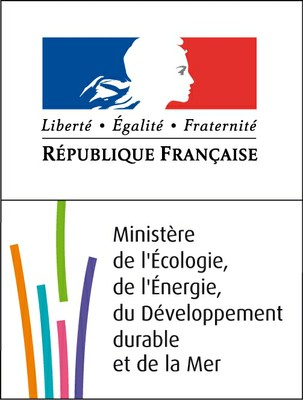Models and numerical methods

Recent advances show the ability to model and simulate free surface complex flows using a conservation law formulation of the fluid equations combined with a kinetic approach. Those results represent an extension of previous studies carried out on the shallow water system or in gas dynamics. In comparison with other classical approaches, we do not use here the parabolic property of Navier Stokes equation. Moreover, we need not use time splitting schemes, nor moving meshes. We start indeed with Euler equations and are able to prove stability, positivity, conservativity, a maximum principle and eventually we end up with balanced schemes.

First tool: a multilayer Saint-Venant system

Incompressible hydrostatic Euler equations with free surface admit the following formulation: \begin{equation} \left\{\begin{array}{l} \frac{\partial u}{\partial x }+\frac{\partial w }{\partial z } =0,\\ \frac{\partial u}{\partial t } + \frac{\partial u^2}{\partial x } +\frac{\partial uw }{\partial z }+ \frac{\partial p}{\partial x } =0,\\ \frac{\partial p}{\partial z } = -g, \end{array}\right. \label{eq:euler} \end{equation} with the free surface boundary condition \begin{equation} \label{eq:free_surf} \frac{\partial \eta}{\partial t} + u_s\frac{\partial \eta}{\partial x} - w_s =0. \end{equation} The process to obtain the multilayer system is described below. It is basically a $$\mathbb{P_0}$$ Galerkin approximation of the variables followed by a vertical integration of the equations. The interval $$[z_b,\eta]$$ is divided into $$N$$ layers $$\{L_\alpha\}_{\alpha\in\{1,\ldots,N\}}$$ of thickness $$l_\alpha H(x,t)$$ where each layer $$L_\alpha$$ corresponds to the points satisfying $$z \in L_\alpha(x,t) = [z_{\alpha-1/2},z_{\alpha+1/2}]$$ with \begin{equation} \left\{\begin{array}{l} z_{\alpha+1/2}(x,t) = z_b(x,t) + \sum_{j=1}^\alpha l_j H(x,t),\\ h_\alpha(x,t) = z_{\alpha+1/2}(x,t) - z_{\alpha-1/2}(x,t)=l_\alpha H(x,t), \quad \alpha\in [0,\ldots,N] \end{array}\right. \label{eq:layer} \end{equation} with $$l_j>0, \quad \sum_{j=1}^N l_j=1$$. Hence, system \eqref{eq:euler} is approximated by \begin{equation} \left\{\begin{array}{l} \frac{\partial H}{\partial t} + \sum_{\alpha=1}^N \frac{\partial h_\alpha u_\alpha}{\partial x} = 0\\ \frac{\partial h_\alpha u_\alpha}{\partial t} + \frac{\partial}{\partial x}\left(h_\alpha u_\alpha^2 + \frac{g}{2l_\alpha} h_\alpha^2\right) = -gh_\alpha\frac{\partial z_b}{\partial x} +u_{\alpha+1/2}G_{\alpha+1/2} -u_{\alpha-1/2}G_{\alpha-1/2} \end{array}\right. \label{eq:msv} \end{equation} The $$u_\alpha$$ are the components of the vertical discretization of the horizontal velocity $$u$$ on the $$\mathbb{P_0}$$ Galerkin basis. The $$G_{\alpha+1/2}$$ are defined by \begin{equation} G_{\alpha+1/2}=\frac{\partial }{\partial t }\sum_{j=1}^\alpha h_j + \frac{\partial }{\partial x }\sum_{j=1}^\alpha h_ju_j, \qquad \alpha=1,\ldots,N. \label{eq:Q} \end{equation} For classical solutions, an equation on energy conservation is added to \eqref{eq:msv} \begin{align} \frac{\partial }{\partial t} \left( \sum_{\alpha=1}^N E_{\alpha}\right) + \frac{\partial}{\partial x} \left(\sum_{\alpha=1}^N u_\alpha\left(E_{\alpha} + \frac{g}{2l_\alpha}h_\alpha^2\right) \right) = 0,\label{eq:energy_mc} \end{align} with $E_{\alpha}=\frac{h_\alpha u_\alpha^2}{2}+\frac{gh_\alpha(\eta+z_b)}{2}.$ Notice that \eqref{eq:energy_mc} is exactly a vertical semi-discretization of the energy balance equation associated to \eqref{eq:euler} on a $$\mathbb{P_0}$$ Galerkin basis. We can adapt the previous method in 3d but also in the case of variable density or in the presence of non hydrostatic effects. Then, the density $$\rho=\rho(T)$$ of the fluid is a function of a given tracer (the water temperature $$T$$ for instance ): \begin{equation} \left\{\begin{array}{l} \frac{\partial u}{\partial x }+\frac{\partial w }{\partial z } =0\\ \frac{\partial u}{\partial t } + \frac{\partial u^2}{\partial x } +\frac{\partial uw }{\partial z }+ \frac{1}{\rho}\frac{\partial p}{\partial x } =0\\ \frac{\partial w}{\partial t } + \frac{\partial uw}{\partial x } +\frac{\partial w^2 }{\partial z } + \frac{1}{\rho}\frac{\partial p}{\partial z } = -g \\ \frac{\partial \rho T}{\partial t}+\frac{\partial \rho u T}{\partial x}+\frac{\partial \rho w T }{\partial z} = \mu_T\frac{\partial^2 T}{\partial x^2} + \mu_T\frac{\partial^2 T}{\partial z^2}. \end{array}\right. \end{equation}
For a better transcription of the physical phenomena we are interested in, several improvements are possible in the equations formulation

The kinetic approach consists in linking the behaviour of some macroscopic fluid systems - Euler or Navier-Stokes equations, Saint-Venant system - with Boltzmann type kinetic equations. The first step is the introduction of fictitious particles, the definition of a density of particles and the equation governing its evolution. For a given layer $$\alpha$$, a distribution function $$M_\alpha(x,t,\xi)$$ of fictitious particles with microscopic velocity $$\xi$$ is introduced to obtain a linear kinetic equation equivalent to the macroscopic model. What is important is that the equation on the density function $$M_\alpha(x,t,\xi)$$ is linear and allows an easy space discretization following classical upwind schemes.